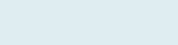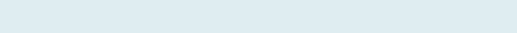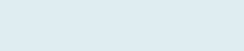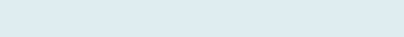# Count Based on Condition with Eight Examples

If you are looking to create a formula to count in Excel IF one or more criteria is true. This article would then help as you understand examples that can guide creating an Excel formula that only counts If matches based on the given condition of text or value.

In these examples, we will consider Excel functions COUNTIF and COUNTIFS with four different lessons of each.

## How many times certain characters appear

But before going to Examples, let’s understand what is COUNTIF Function.

The Excel COUNTIF function returns a count of numbers based on given criteria.Now, let’s work around three Excel COUNTIF’s example on a worksheet.

Remember: You can also evaluate the formula using a shortcut F9 function key or under the formulas, tab click on Evaluate formula.Note: :  ‘&’ operator in Excel is a type of concatenate which is used to join two strings, values or functions.

## Count Negative Numbers

After reading this article you will be able to count negative numbers and zeros  excluding positive numbers.

Query: In this example, we have two columns of Student and Marks. Our task is to know how many student get negative marks.

Formula to implement:

=COUNTIF(C3:C12,”<0″)

Result: 3

It first checks the given number in the range (C3 to C12) as 3 Student marks are negative(in column C) so, it returns 3 ignoring positive numbers.

Remember: Same way you can give count of positive number instead “<0” put “>0” in Formula.

## Count Cells Excluding Error

When you have a column where some cells are filled with error and remaining with text. To count text after excluding #NA value.

Query: In this example, we have two columns of Name and Department. Our task is to know how many people are still working in each department.

Formula to implement:

=COUNTIF(C3:C21,”<>#N/A”)

Result: 15

It returns counts in the given range (C3 to C21) after excluding #N/A values in the cells.

Note: COUNTIF is a combination of COUNT and IF Excel functions.

## Count occurrence of text in range

In this article, you are going to learn how to count cells with repetitive Text. In case you want to show text with the number of occurrences.

Query: In this example, we have two columns of Items and Count. Our task is to know how many times similar items come in multiple rows to give the cumulative number in column C.

Formula to implement:

=COUNTIF(\$B\$3:B3,B3)&””&B3

Result: 1 Duck Tape

(Count = 1)

It counts based on criteria (B3) then return text with count (1 Duck Tape) in column C (Count).

Similarly, in column C it will return the count of each item with Name. like in cell C6 it returns (2 Duck Tape) because Duck Tape comes two times.

Remember: Please go to how to count only Numbers or Text in a column.

## Count Duplicates values in rows

To give the count of the column with two different criteria then later add them by counting how many times they (both) come in the range.

Query: In this example, we have two columns of Food items and Payment Mode. Our task is to know how many Food items have been paid.

Formula to implement:

=COUNTIF(C3:C13,C3)+COUNTIF(C3:C13,C4)

Result: 7It will first check both criteria (Cash and Online) and then give the count of both (4 and 3) in the given range (C3 to C13) and then add them.

Note: You can use COUNTIF function to count when there are two different texts in the same range.

Now let’s understand what is COUNTIFS function in Excel before going with four examples.

The Excel COUNTIFS function returns a count of numbers based on one or multiple given criteria. COUNTIFS can be used to count values when selected cells meet criteria based on dates, numbers, and text.

The COUNTIFS has the following arguments:Now, let’s see how we can use the Excel COUNTIFS function on the real-time worksheet to find a count of cells that meet all of the criteria.

## Number of Days Between Dates

In case you want to know how many days between two dates, in the array to get the count.

Query: In this example, we have two columns of Date and Code. Our task is to know how many days are greater than or equals to start date and less than or equals to the end date.

Formula to implement:

=COUNTIFS(B:B,”>=”&\$E\$8,B:B,”<=”&\$E\$9)Counting days greater or equal to start date and less and equal to end date.

Result: 20

It checks both criteria (“>=” and “<=”) then gives the count of dates which are greater than equals to start date but less than or equals to end dates.

Note: COUNTIFS is a combination of COUNT and IFS Excel functions.

## Count if Text Matches next Column

In case there is matching text in both columns and you want to count only a specific text of both in the range.

Query: In this example, we have two columns of Region and Item Category. Our task to count the only Region with North and Item Category with Office Supplies.

Formula to implement:

=COUNTIFS(B3:B21,B4,C3:C21,C4)

Result: 4It will first check both criteria North (in column B) in the range (B3 to B21) and Office Supplies (in column C) in the given range (C3 to C21) to give count.

## Count with more than two Condition

When you have multiple criteria and to know count based on different rows or columns (greater or less than).

Query: In this example, we have three columns of Order, Quantity, and Price. Our task is to get a count of Order “Burger” whose Quantity is less than 10 and Price is less than 100.

Formula to implement:

=COUNTIFS(B3:B18,B4,C3:C18,”<10″,D3:D18,”<100″)Count of burger with quantity and price less than 10 and 100 respectively.

Result: 2

It first checks three criteria order of Burger (in column B) with Quantity less than 10 (in column C) and Price less than 100 (in column C) in the given range to give count.

Note: COUNTIFS can check up to 20 criteria with criteria range.

## How many times certain characters appear

Counting characters match with certain start and end letters. For this, we use a wildcard symbol (*) asterisk that gives us enough flexibility to returns the results that are alike.

Query: In this example, we have two columns of Furniture and Quantity. Our task is to count furniture which starts with character C and ends with character S.

Formula to implement:

=COUNTIFS(\$B\$3:\$B\$13,”C*”,\$B\$3:\$B\$13,”*S”)

Result: 3

It will count furniture that starts with character C and end character S in the given range (B3 to B13).

You can also learn how to Sum, and Average based on condition with examples.